Next: Moment of inertia Up: Rotational motion Previous: The vector product

## Centre of mass

The centre of mass--or centre of gravity--of an extended object is defined in much the same manner as we earlier defined the centre of mass of a set of mutually interacting point mass objects--see Sect. 6.3. To be more exact, the coordinates of the centre of mass of an extended object are the mass weighted averages of the coordinates of the elements which make up that object. Thus, if the object has net mass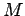, and is composed ofelements, such that the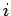th element has mass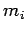and position vector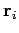, then the position vector of the centre of mass is given by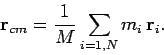(325)

If the object under consideration is continuous, then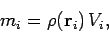(326)

where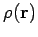is the mass density of the object, and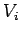is the volume occupied by theth element. Here, it is assumed that this volume is small compared to the total volume of the object. Taking the limit that the number of elements goes to infinity, and the volume of each element goes to zero, Eqs. (325) and (326) yield the following integral formula for the position vector of the centre of mass: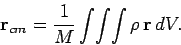(327)

Here, the integral is taken over the whole volume of the object, and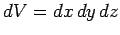is an element of that volume. Incidentally, the triple integral sign indicates a volume integral: i.e., a simultaneous integral over three independent Cartesian coordinates. Finally, for an object whose mass density is constant--which is the only type of object that we shall be considering in this course--the above expression reduces to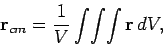(328)

whereis the volume of the object. According to Eq. (328), the centre of mass of a body of uniform density is located at the geometric centre of that body.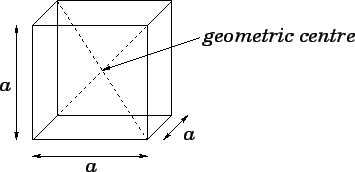For many solid objects, the location of the geometric centre follows from symmetry. For instance, the geometric centre of a cube is the point of intersection of the cube's diagonals. See Fig. 72. Likewise, the geometric centre of a right cylinder is located on the axis, half-way up the cylinder. See Fig. 73.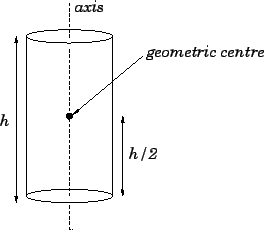As an illustration of the use of formula (328), let us calculate the geometric centre of a regular square-sided pyramid. Figure 74 shows such a pyramid. Letbe the length of each side. It follows, from simple trigonometry, that the height of the pyramid is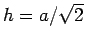. Suppose that the base of the pyramid lies on the-plane, and the apex is aligned with the-axis, as shown in the diagram. It follows, from symmetry, that the geometric centre of the pyramid lies on the-axis. It only remains to calculate the perpendicular distance,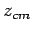, between the geometric centre and the base of the pyramid. This quantity is obtained from the-component of Eq. (328):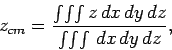(329)

where the integral is taken over the volume of the pyramid.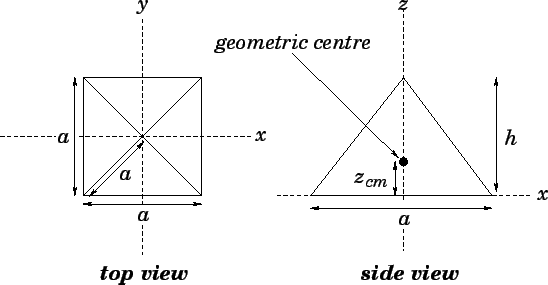In the above integral, the limits of integration forare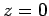to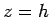, respectively (i.e., from the base to the apex of the pyramid). The corresponding limits of integration forandare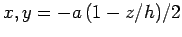to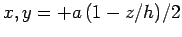, respectively (i.e., the limits are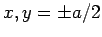at the base of the pyramid, and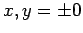at the apex). Hence, Eq. (329) can be written more explicitly as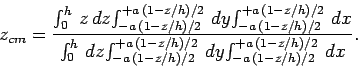(330)

As indicated above, it makes sense to perform the- and- integrals before the-integrals, since the limits of integration for the- and- integrals are-dependent. Performing the-integrals, we obtain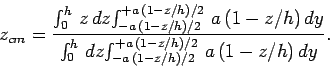(331)

Performing the-integrals, we obtain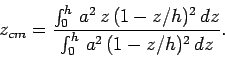(332)

Finally, performing the-integrals, we obtain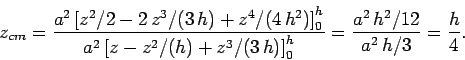(333)

Thus, the geometric centre of a regular square-sided pyramid is located on the symmetry axis, one quarter of the way from the base to the apex.Next: Moment of inertia Up: Rotational motion Previous: The vector product
Richard Fitzpatrick 2006-02-02